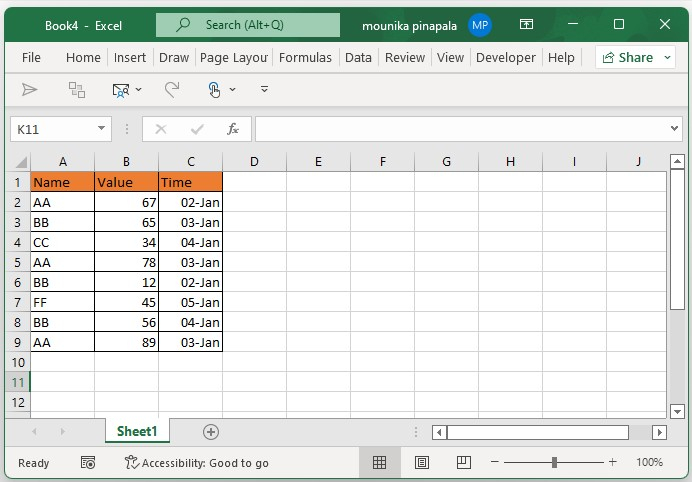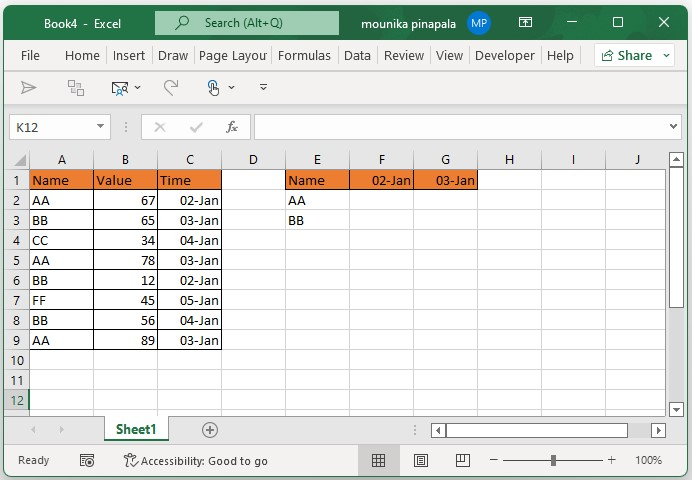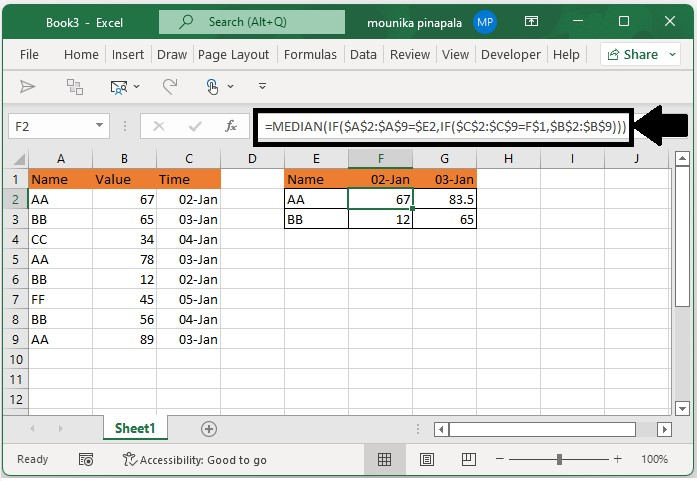# How to calculate the median with multiple conditions in Excel?

The calculation of the median in a specific range may be easy for you, but if you ever wish to calculate the median in a Microsoft Excel sheet that has multiple conditions, kindly follow the below steps to understand it easily. In this article, you will learn how to calculate the median if multiple conditions are in the Microsoft Excel sheet.

Consider an example that you have a specific range of data, and you want to calculate the median value of 2-Jan. To calculate the median that meets this condition, follow the steps given below.

## Step 1

Open a Microsoft excel sheet and enter the data of names, values, and time as shown in the below screenshot for your reference.## Step 2

In a given range, assume that you want to calculate the median for only 2 Jan and 3 Jan. you can mentioned this information in Microsoft excel sheet as shown in below screenshot for your reference.## Step 3

Now you need to enter the below given formula in cell F2 and press enter key or you can click on Shift + ctrl + Enter key at the same time and then you need to drag the fill handle to fill the range with the given formula. Kindly find the below screenshot for your reference.

=MEDIAN(IF($A$2:$A$9=$E2,IF($C$2:$C$9=F$1,$B$2:$B$9)))In the above screenshot, you can find the median values for 2 Jan and 3 Jan for the name AA and the name BB.

## Conclusion

In this tutorial, we used a simple example to demonstrate how you can calculate the median in Excel if multiple conditions are given.